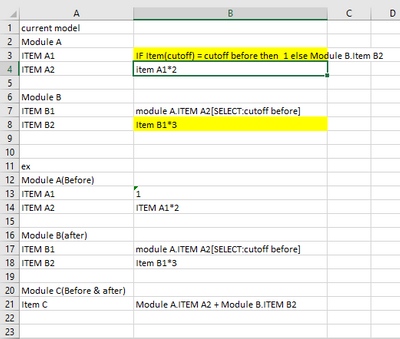# Avoid circular reference using list member logicHi all,

I’m new to Anaplan and would really appreciate help with the following:

I'm trying use the same module ('Module A') for two stages of a calculation (before and after); for this, 'Module A' has only one dimension; a list or two members ('Before' and 'After'), image attached. it's first line item (Item A1) sets a fixed value for the 'Before' list member and should set a reference to 'Module B' for the 'After' list member. Right now it only sets to zero in the attached image because of the circular reference, if the circular reference could be resolved it would be:

IF ITEM(Cutoff) = Cutoff.Before THEN 1 ELSE Module B.'Item B2'

'Module B' has no dimensions, and its first line item takes the second line item of 'Module A', selecting list member 'Before'. Image attached.

As I mentioned, if I apply the formula above, i get a circular reference. I understand the reason of the circular refence because of the line items involved, but I wanted to know if this can be avoided by selecting the proper list members in the calculations; as you can see, logically, I'm not incurring in a circular reference, since I'm first doing the 'Before' calculations and later the 'After', even though the line items are the same.

I understand this is a matter of what Anaplan is designed to do; if it will get a circular reference even if the logic is sound list member wise, there is nothing I can do, just wanted to know if that is the case or if there is a way to avoid the circular reference through list member logic, even if the line items are incurring in circular reference.

Any help would be greatly appreciated.

Thanks in advance for any help and best regards,

Wolfgang

Tagged:

•Hi wolfgang,

why get the circular reference because calculation in your model using same item, in model B to get data Item B2, you take data form model A, and in model A to get data after you take data from model B, it will be circular. the solution is you need to break model A and model B using subset, and you need create model C to combine beetween model A and model BRegards,

Panji

•An alternative solution would be to use an action to import the value from one module into the other only when the result of the IF THEN ELSE is TRUE.

This would break the circular reference and allow you to transfer the values between modules.

The circular reference is due to the use of the common dimension. From the screenshots you have provided the list is redundant and the same outcome could be achieve if you simply used line items.

•Thanks all for the replies, specially so promptly and in detail. In the end I will go with Nathan's suggestion; I saw that workaround, but seemed a little too complex for me at the time.

Thanks all for clarifying this for me.

Best regards,

Wolfgang